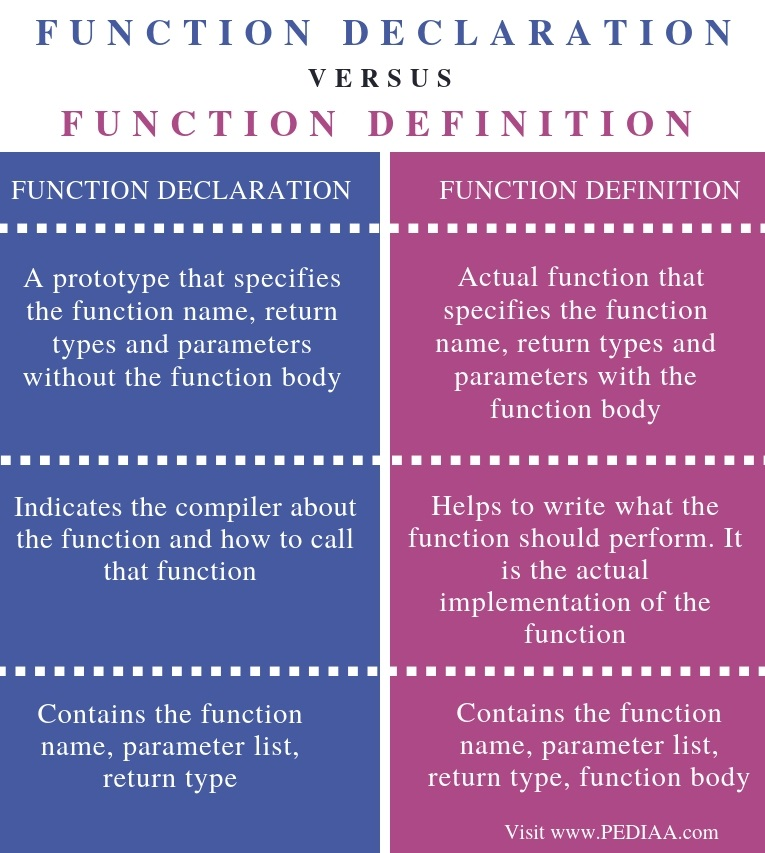# What is the Difference Between Function Declaration and Function Definition in C Programming

The main difference between Function Declaration and Function Definition in C Programming is that Function declaration indicates what the function is and Function Definition indicates what the function does.

C is a high-level general purpose programming language developed by Dennis Richie. It is the foundation programming language of many other languages such as C++, Python, Java, PHP, etc. A function is an important concept in C programming. It is a set of statements that performs a particular task. Rather than writing all the statements inside the main program, the programmer can create functions and call them within the main program. It makes the program more readable. After the execution of the function, the control passes back to the main program. A function has a declaration and definition. The declaration is a prototype whhile the definition contains the actual implementation.

### Key Areas Covered

1. What is Function Declaration in C Programming
– Definition, Functionality
2. What is Function Definition in C Programming
– Definition, Functionality
3. Difference Between Function Declaration and Function Definition in C Programming
– Comparison of Key Differences

### Key Terms

C Programming, Function Declaration, Function Definition## What is Function Declaration in C Programming

Function declaration indicates the compiler about a specific function. The syntax is as follows.

return_type function_name (parameter list);

The function_name helps to identify a function. The parameter list consist of what is passed to the function to perform the task. The return_type refers to the data type of the output of the function. If the function gives an integer, the return type is int. If it is a double value, the return type is double. If the function does not return any value, then it is a void.

For example, assume a function that multiplies two integers. The function declaration is as follows.

int multiply (int num1, int num2);

The function is called multiply. It gets two integers called num1 and num2. The return type is int. Therefore, the function outputs an integer value. Finally, the declaration ends with a semicolon.

It is also possible to mention only the data types of the parameters in the declaration as follows.

int multiply (int, int);

## What is Function Definition in C Programming

Function definition refers to the implementation of the function. In other words, it represents the actual statements that the function performs. The syntax is as follows.

return_type function_name (parameter_list){

// statements of the function

}

The function_name helps to identify the function. When invoking the function, the values pass to that function. These values copy to the parameters. There can be single or multiple parameters with their corresponding data types. The return type explains the output of the function. The programmer can write the statements of the function inside the curly braces.

An example program is as follows.Figure 1 : C program with function declaration and definition

In the above program, line 3 displays the function declaration. It provides information about the function to the compiler such as name, parameters, etc. In the main method, there are two integers: num1 and num2. In line 9, these values are passed to multiply. The function is executed. The num1 value copies to x and num2 value copies to y. Then the result is returned and stored to the variable ans. Finally, the printf statement displays the value on the console.

In the above program, line 3 displays the function declaration whereas line 14 to line 16 displays the function definition.

## Difference Between Function Declaration and Function Definition in C Programming

### Definition

Function declaration is a prototype that specifies the function name, return types and parameters without the function body. Function Definition, on the other hand, refers to the actual function that specifies the function name, return types and parameters with the function body. Thus, this is the main difference between function declaration and function definition.

### Functionality

Functionally, the difference between function declaration and function definition is that the function declaration helps to indicate the compiler about the function and how to call that function, etc. while Function definition helps to write what the function should perform. It is the actual implementation of the function.

### Consist of

Function declaration contains the function name, parameter list, and, return type while Function definition contains the function name, parameter list, return type, function body (statements of the function). This is another difference between function declaration and function definition.

### Conclusion

In C programs, the function has a declaration and a definition. The main difference between function declaration and function definition in C Programming is that Function declaration indicates what the function is and Function Definition indicates what the function does.

##### Reference:

1.“Functions in C – Javatpoint.” Www.javatpoint.com, Available here